Tutorial math and reading software for elementary and secondary arithmetic, basic math, algebra, geometry, precalculus plus GED, ABE, and CLEP preparation for elementary school, high school, college, adult education, and homeschool students.

# Algebra help comes in a bundle price forthe second semester set of algebra software(Chapters 5 - 8)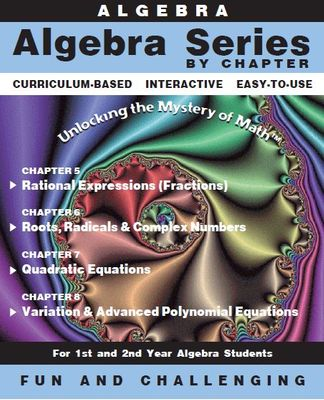Algebra Software By Chapter
2nd Semester Bundle (Ch 5 - 8)
Item# Alg-Ch5-8
Regular price: \$159.00
Limited Time Offer: \$79.50
Media type::

## Product Description

The second semester set of this algebra software is appropriate for second semester algebra 1 students, first semester algebra 2 students & college algebra students. Algebra help is available from each practice question.

Chapter 5: Rational Expressions (Algebraic Fractions)
Performing operations of addition, subtraction, multiplication, and division on fractions is always a challenging topic for students. This program reviews the basics operations on numerical fractions before advancing to operations on algebraic fractions. Also, included in this program are negative powers and scientific notation. The study of algebraic fractions concludes with a word problem section using the learned material from the body of the program.

Chapter 6: Roots, Radicals, and Complex Numbers
Follow the progression from simplifying roots and radicals, through the properties of radicals. Practice sums of radicals and learn about binomials by rationalizing denominators and applying FOIL (multiplication of binomials). Solving radical equations are practiced. A thorough discussion and practice involving rational and irrational numbers is followed by an in-depth study of imaginary and complex numbers. The problem solving section and test conclude this program.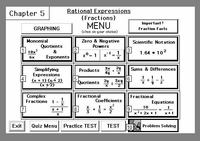Chapter 5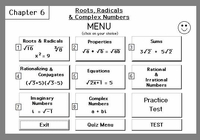Chapter 6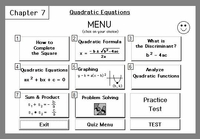Chapter 7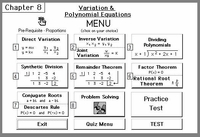Chapter 8